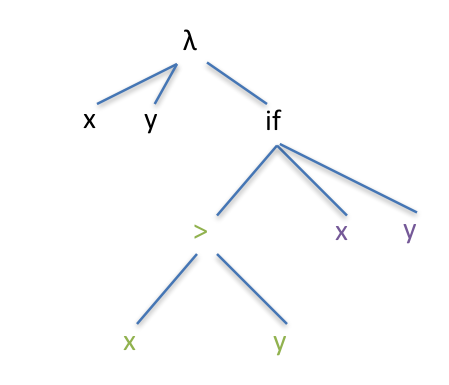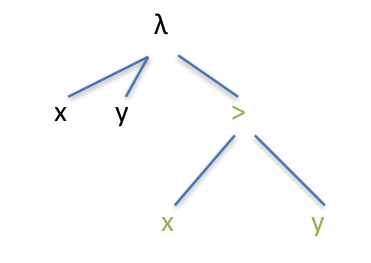# Boolean and Relational Operators

Boolean operators are operators such as and, or and not, and specifically deal with logic values. For example, (TRUE AND TRUE) evaluates to TRUE, while (TRUE AND FALSE) is FALSE. Relational operators, on the other hand, are comparison operators, things like <>, etc. These compare two or more items and return a Boolean value to indicate whether or not the relation is TRUE. For example ( 2 > 1) is TRUE, while (1 > 2) is FALSE.

In prefix notation, as you would expect, we simply put the operator first:

• (> 2 1)..True
• (< 4 2) .. False

In Racket, we use #t and #f for TRUE and FALSE, so we could have

```> (> 2 1)
#t
> (= 2 1)
#f
> (< (+ 3 1) (* 4 5))
#t
```

Notice in the third example we have a mix of arithmetic and relational operators. This is okay as long as their order makes sense; in that third example, the arithmetic operations return 4 and 20, respectively, which are perfectly legal to pass to <. However, if we tried this (+ 2 (> 3 1)) we would get an error, because is not defined for operating on a Boolean value, that is, (+ 2 #t) doesn’t really make sense.

Where we want to use these sorts of logic values is in conditionals, specifically in the predicate for a conditional, which is the logic test performed in something like an IF statement. A general view of a conditional is something like

if E then C1 else C2

That is, if the predicate in expression  is true, then execute C1, otherwise execute C2. The λ calculus and Racket view of things is similar:

if E then RETURN C1 else RETURN C2

Why do we say RETURN? Because typically we’re dealing with expressions, so, depending on the result of the test in E, we return the value in either C1 or C2.

Let’s look at some examples. Here’s a conditional in Racket:

```> (if (> 2 0) "first" "second")
"first"
```

That is, if 2 is greater than 0, return the word “first”, otherwise return the word “second”. Pretty straightforward… so far!

Here’s a λ calculus expression:

(λxy. if (> x y) x y)

This takes two parameters and returns the first if it is larger, and otherwise returns the second.A simple if construct in lambda calculus. The part in green is the predicate, which returns a logic value of true or false.

If we get a true value in the predicate, the AST returns x, otherwise it returns y.

Here’s a similar but different one:

(λxy. > x y)

Notice that this one doesn’t even have an IF part, it just performs a logic test and returns the value of that — either true or false.A lambda expression that returns a Boolean value, but which doesn’t use an if. Notice that it simply returns the result of the > test.

λ calculus can use all the classical Boolean constructs, such as and, or, not etc. The next section will look at these and highlight some areas that might be surprising to people who are used to programming with other languages.